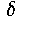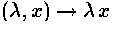# Noncommutative Geometry, Trace Formulas and the Zeros of the Riemann Zeta Function

### Abstract

In this course we first give a general introduction to noncommutative geometry. We then discuss a fundamental example of noncommutative space related to the Riemann zeta function. This gives a spectral interpretation of the critical zeros of the Riemann zeta function as an absorption spectrum, while the noncritical zeros appear as resonances, and a geometric interpretation of the explicit formulas of number theory as a trace formula on a noncommutative space. This reduces the Riemann hypothesis to the validity of the trace formula, which remains unproved, and eliminates the parameterof our previous approach.

### Topics

1. Introduction to noncommutative geometry
2. Quantum chaos and the hypothetical Riemann flow.
3. Algebraic geometry and global fields of nonzero characteristic.
4. Spectral interpretation of critical zeros.
5. The distribution trace formula for flows on manifolds.
6. The actionof K* on K for a local field.
7. The global case, and the formal trace computation.
8. The trace formula and S-units.
9. The trace formula in the global case, and elimination of.
10. Explicit formulas.

### Noncommutative Geometry and the Riemann Zeta Function

Alain Connes' expository paper on the subject matter of the course, describing his motivations and some background. It is available for downloading in any of the following formats:

LaTeX, DVI, PostScript, PDF (Adobe Acrobat v. 3.0 or later)

### Trace Formula in Noncommutative Geometry and the Zeros of the Riemann Zeta Function

This is Alain Connes' latest paper, on the subject matter of this course. It is available for downloading in any of the following formats:

Plain TeX, DVI, PostScript, PDF (Adobe Acrobat v. 3.0 or later)

### Registration Information

The current listing for Alain Connes' course is

 MATH 975A TOPICS IN GEOMETRY 3 CR Section: 18473-7 MWF 2:30-4:20PM CL 0220
The course will run for 5 weeks and the tentative dates are
Oct. 12 - Nov. 13

### Update

Update4: Lectures on M (Nov. 9) and F (Nov. 13) during the fifth week

The first lecture of the course will be Wed. Oct. 14. The second lecture will be Fri. Oct. 16. The lectures will be held MW the second week, WF the third week, and MW the fourth week. All lectures will be at 2:30-4:20 pm, in room CL 0220. (CL is Caldwell Laboratory, 2024 Neil Ave. Click here for a map.)